Courses

# Test: Electrical Machines- 7

## 10 Questions MCQ Test RRB JE for Electrical Engineering | Test: Electrical Machines- 7

Description
This mock test of Test: Electrical Machines- 7 for Electrical Engineering (EE) helps you for every Electrical Engineering (EE) entrance exam. This contains 10 Multiple Choice Questions for Electrical Engineering (EE) Test: Electrical Machines- 7 (mcq) to study with solutions a complete question bank. The solved questions answers in this Test: Electrical Machines- 7 quiz give you a good mix of easy questions and tough questions. Electrical Engineering (EE) students definitely take this Test: Electrical Machines- 7 exercise for a better result in the exam. You can find other Test: Electrical Machines- 7 extra questions, long questions & short questions for Electrical Engineering (EE) on EduRev as well by searching above.
*Answer can only contain numeric values
QUESTION: 1

### A synchronous generator has synchronous reactance of 1.5 PU. It is connected to an infinite bus voltage 1 PU, equivalent reactance of 0.4 PU. If the maximum output is 2 PU, the load angle for an output of 1 PU is _______ degrees.

Solution:

P = Pmax sin δ
1 = 2 sin δ
δ = 30°

*Answer can only contain numeric values
QUESTION: 2

### A 12 pole 3ϕ alternator driven at speed of 500 rpm supplies power to an 8 pole, 3ϕ I.M. If the slip of the motor at full load is 3%. Calculate full load speed of the motor.

Solution:

For alternator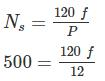F = 50 Hz
For I.M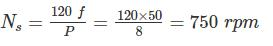Nr = (1 - S) Ns = (1 - .03) × 750
∴ Nr = 727.5 RPM

QUESTION: 3

### At a location in India, it is necessary to supply 300 kW of 60 Hz power. The only power source available operate at 50 Hz. It is decide to generate the power by means of a motor-generator set consisting of a synchronous motor driving synchronous generator. How many pole should each of the two machine have in order to convert 50 Hz power to 60-Hz power?

Solution: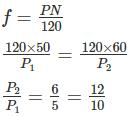∴ A 10-pole synchronous motor must be coupled to a 12-pole synchronous generator to accomplish this frequency conversion.

QUESTION: 4

A synchronous generator connected to an Infinite bus is under excited. Considering only the reactive power, from the point of the system the machine act as

Solution:

For generator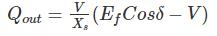When Ef cosδ < V, i.e under excited generator, then reactive power (Qout) is negative i.e supplying leading VARs and therefore operating at leading power factor, thus behaving like a capacitor.

*Answer can only contain numeric values
QUESTION: 5

A 30 KVA, 230 V, Y connected, 3 phase salient pale synchronous generator supplies rated load at 0.707 lagging power factor. The reactance per phase are Xd = 2Xq = 4 Ω. Neglect the armature resistance determine the power angle (in degree).

Solution: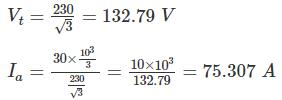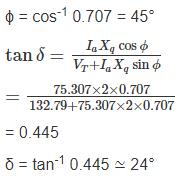QUESTION: 6

A 3 phase alternator has generated emf per phase of 300 V with 15% third harmonic and 10% fifth harmonic content. If the alternator is star connected, what is the value of rms line voltage?

Solution:

In star connection, the third harmonic component of the three phases cancel out at the line terminals because they are co phased.
∴ the line emf will be consisting of the fundamental and fifth harmonic only
Fundamental component E1 = 300 V
5th harmonic component, E5 = 0.1 × 300 = 30 V
Emf per phase,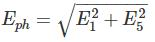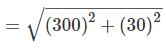= 301.5 V
rms line voltage,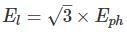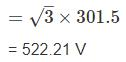*Answer can only contain numeric values
QUESTION: 7

A salient pole synchronous generator has Xof 0.90 PU and Xq of 0.6 PU. The armature resistance is negligible and generator delivers rated KVA at unity P.F and rated terminal voltage. What is the value of excitation voltage?

Solution:

Generated voltage of salient pole synchronous generator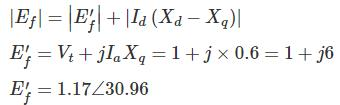Direct component of current
Id = Ia sin 30.96 = 0.5145 PU
∴ |EF| = 1.17 + .5145 × 0.3 = 1.324 PU

*Answer can only contain numeric values
QUESTION: 8

A 4 pole 50 Hz star connected alternator has 8 slots/pole/phase, conductor per slot being 8 and flux per pale 0.28 wb. If the winding coil span is 150°, the inductor phase voltage is ________ V.

Solution:

Given
Slots/pole/phase m = 8
Conductor/slot = 9
Coil/pole = 24
Slot = 24 × 4 = 96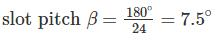Total no. of conductor z = 96 × 8 = 768
Conductor/phase = 256
Number of turn = 128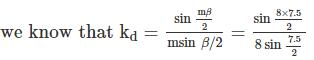= 0.9556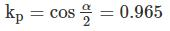Eph = 4.44 × 0.9556 × 0.965 × 0.28 × 50 × 128 = 7337.09 V

QUESTION: 9

An alternator has 18 slots/pole and first coil lies in slots 1 and 16. What is the ratio of pitch factor for 3rd harmonic and 5th harmonic

Solution:

Coil span is 16 – 1 = 15 slots
Number of empty slots = 18 – 15 = 3 slots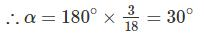Pitch factor for 3rd harmonic,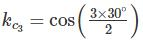= 0.707
Pitch factor for 5th harmonic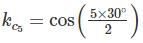= 0.259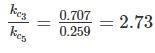*Answer can only contain numeric values
QUESTION: 10

A 30 KVA, 230 V, Y connected, 3 phase salient pale synchronous generator supplies rated load at 0.707 lagging power factor. The reactance per phase are Xd = 2Xq = 4 Ω. Neglect the armature resistance determine The percentage voltage regulation__________

Solution: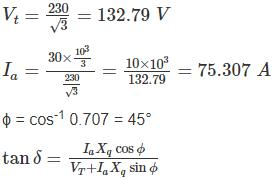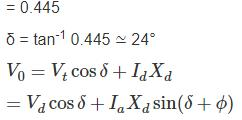= 132.79 × 0.9136 + 75.307 × 4 sin (24 + 45°)
= 121.316 + 281.19
= 402.506
Percentage voltage regulation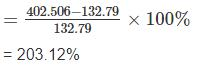Track your progress, build streaks, highlight & save important lessons and more!

### Similar Content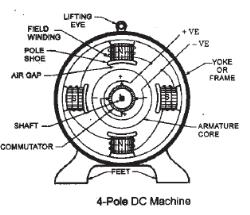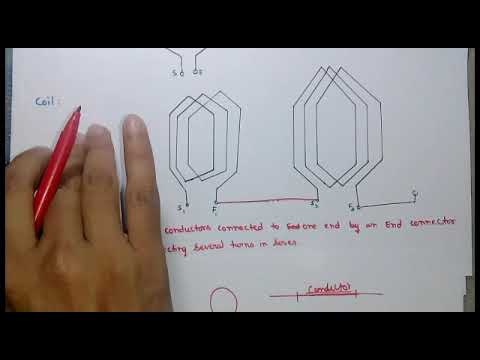### Related tests